### Orlov S*

Petrozavodsk State University, Petrozavodsk, 185001, Russia

Received Date: 07/09/2020; Published Date: 24/11/2020

*Corresponding author: Sergey Orlov, Petrozavodsk State University, Petrozavodsk, 185001, Russia. Email: ion@sampo.ru

### Abstract

A new physical model is proposed for the appearance of an astronomical object - the Black Hole. It is shown that the Black Hole is the center of the cosmic, etheric, gravitational torsion. An equation for determining the radius of this object based on the theory of vortex gravity is presented. A substantiation is proposed that the force of gravity on the surface of the Black Hole does not depend on the mass of this Black Hole. The mass of the Black Hole can not be determined. A contradiction is shown in the Schwarzschild solution by the definition of the radius of the Black Hole.

Keywords: theory of vortex gravitation, celestial mechanics.

### Introduction

According to many astrophysicists, astronomical object the Black Hole (BH) is an area in the space which gravitational attraction is so great that light quanta can't leave it even. Researchers believe that Black holes could result from catastrophic gravitational collapse of a massive star at that historical moment when it dies. At collapse - catastrophic compression of a star - intensity of gravity over its surface becomes so terribly big that the space surrounding a star - time is displaced. This star disappears from the Universe and there is only strongly bent area of space - to time. The border of this area is called as gravitational radius. Spherically a symmetric black hole it is equal in the elementary case to Shvartsshild's radius. Theoretically possibility of existence of such areas of space - time follows from some exact solutions of the equations of Einstein, first of which was received by Karl Shvartsshild in 1915 .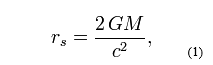Where, rs – the radius of the Black Hole, M – the mass of a black hole, with - the velocity of light, G=6.672 ∙10 -11 N∙m2/kg2 – a gravitational constant. We will consider objectivity of a hypothesis of an origin of the Black hole on the example of similar object which is in the center of our galaxy. In work  the radius of this BH which is called the Sagittarius A * is determined –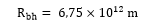At such radius and the corresponding volume, the mass of this BH has to be on the basis of a formula (1) about 1039 kg. Then density of the Black Hole in the center of our galaxy has to be about 5 kg/m3! ? On the basis of the classical equation about a mutual attraction gravitation force on a surface of the Sagittarius A * is equal

F =  6.8 x 104 M

Agree, it is impossible to call this Black hole "catastrophically squeezed", and gravitation forces on its surface  "terribly big". It is obvious that the theoretical explanation of an astronomical phenomenon of the Black hole doesn't correspond to physical parameters. In addition, equation (1) is mathematically absurd. In it, the quantities (G) and (c) are constants. Therefore, the mass of the Black Hole (M) is directly proportional to its radius (r). In fact, the mass of any body is always proportional to its volume. The volume is proportional to the radius of this body in the cube (r3). Then the mass must also be proportional to the radius in the cube. From this discrepancy with the laws of mathematics of equation (1) it follows that the density of the Black Hole (P) is inversely proportional to the root of its cubic radius.
P ~ r-1/3

Example, On the basis of formula (1), we shall determine the mass of the Black Hole with a radius equal to 1 m.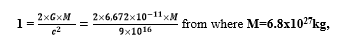Then the density of this black hole is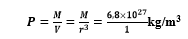This density is several orders of magnitude greater than the density of the nucleon. Consequently, equation (1) is absurd. It is offered to study the theory of vortex gravitation and cosmology below. On the basis of this theory it is possible to explain genesis of the Black hole without contradictions.

### About the Theory of Vortex Gravitation

The theory of vortex gravitation  is based on the well-known astronomical fact – all heavenly objects rotate. The most logical explanation of the reason of this movement can be only one – rotation of heavenly objects generated vortex rotation of space substance – ether. Ether forms system of the interconnected whirlwinds in world space. Orbital speeds of ether in each tuft (torsion) decrease in the direction from the center to the periphery under the law of the return square of this removal.  If orbital speeds of a stream of ether decrease then, under aerodynamics laws, pressure in this stream increases. The gradient of pressure generates pushing out force in the direction to zones with the smallest pressure, that is to the center of this torsion. We will consider the equation of vortex gravitation received in the theory . In this section, a model of appearance of the gravitation attraction force is considered from the viewpoint of aerodynamics. Namely, the two-dimensional model is considered on the basis of the following initial postulates. These postulates will be expanded and defined more exactly below.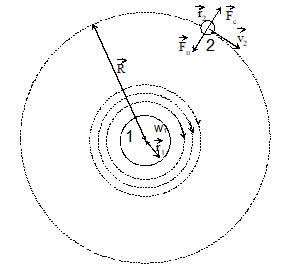Figure 1: Two-dimensional model of gravitational interaction of two bodies.

Forces operating on a body 2 are specified. The Fc-centrifugal force, Fp-force of an attraction of a body 2 from a body 1, v2 – the linear speed of a body 2 on an orbit, R – the radius of an orbit, r1 – the radius of a body 1, r2 – the radius of a body 2, w1 – the angular speed of rotation of air on a surface of a body 1, m2 – body weight 2.

As it was already spoken, movement of a whirlwind is resulted by pressure gradient. Radial distribution of pressure and ether speed in work  are defined on the basis of Navier-Stokes's equation for movement of viscous liquid (gas).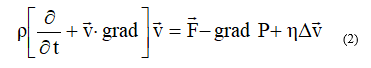In cylindrical coordinates taking into account radial symmetry of vr=vz=0, vj=v (r), P=P (r) the equation will register in the form of system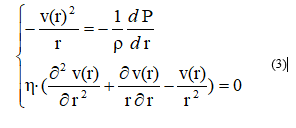Where r = 8.85 х 10-12  kg \m3 - density of ether , – a vector of speed of ether, P – pressure of ether, h- viscosity.

In cylindrical coordinates for the module of force of gravitation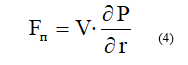Then comparing (3) and (4) for incompressible ether (r=const) we find that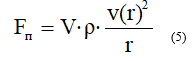After necessary transformations (full calculation is stated in the theory ) it is received:

1 . the equations for determination of force of gravitation depending on the speed of rotation of ether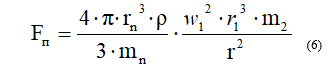rn,  mn  – radius and mass of a nucleon.

We will transform a formula (6). We will equate r1 = r. We will substitute w1 r1 = v1 and numerical values rn,  mn, r, we receive:2. The equations for determination of dependence of pressure of P0, from the speed of rotation of ether of V1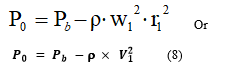Where – pressure of ether on we consider P0 to an orbit, using a boundary condition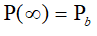In fig. 2 pressure distribution according to a formula (8) is graphically shown.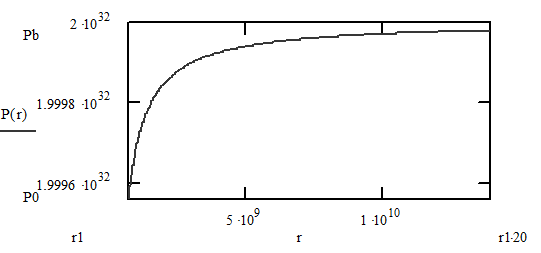Figure 2: Radial distribution of pressure of air for the Sun.

### Vortex Black Hole

Асcording to laws ether-loudspeakers  pressure in motionless air is accepted size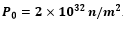Ether density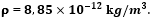By means of the equation (8) we will find the orbital speed of ether of V0 = V1 at which pressure of P0 will be equal to zero.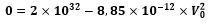from where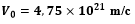Orbital speed of ether to belong to the radius of the orbit under the law of the return square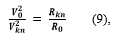where

R0  – radius of an orbit of a whirlwind on which ether reaches speed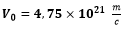Rkn – radius of an orbit of a whirlwind in which the speed of ether is known (Vkn).

From the equation (9) we find the orbital radius of the radio torsion with zero pressure.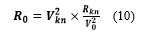Orbital speed of ether of Vkn is determined by the known force of gravitation in the same orbit, by means of the equation (7).

### Radius of the Vortex Black Hole of the Sagittarius*

We will consider the Sun address in a galaxy orbit.

Orbital speed of solar system -V = 2.2 * 105

Radius of an orbit of solar system - Vkn = 2.46*1020 .

We determine the centrifugal force operating on the Sun.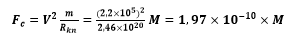Provided that centrifugal forces, in any point of an orbit, are always equal to attraction forces, we find force of the galactic gravitation operating on the Sun.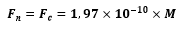We substitute value Fп and r in the equation (7) and we find ether speed in a solar orbit.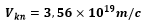Radius of an orbit of astronomical object the Sagittarius* with the zero pressure upon surfaces, on the basis of the equation (10)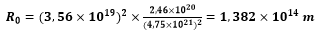The strength of the vortex gravitation on the surface of a black hole

Fg = 620 x M

Similarly, we find radiuses of Black holes at other objects:

Sun - R0 = 155500 m

Eartht - R0 = 0.478 m

### Conclusion

Obviously, the modern theory of the origin of black holes contradictory. On the example of a celestial object Sagittarius A* can be argued that Black holes are not superdense and supermassive objects. They may not have a huge force of gravity at its surface.  Based on the theory of vortex gravitation and cosmology, black holes is the central region of space, ether, gravitation torsion. Orbital velocity of the ether on the surface of this area reaches the maximum possible value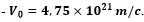At this speed, the pressure on the rotationorbitof theesterdecreases to zero. No substanceorradiation is notable tobreak out ofthis zone.Therefore, the centerspace, gravitation torsioninvisible.Insidethe black holerotationetherorstopsorslows down. In this case,there can existinside a black holeof antigravity.

Note. A similar phenomenon is noted in the center of tropical meteorology and sea storms. Where there is complete peace of mind (calm). This phenomenon is called the "eye of the storm." Radius of the black hole Sagittarius A * at the center of our galaxy observations to determine the magnitude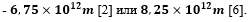The estimated value of the radius of the black hole Sagittarius A *, obtained in Chapter 4 (1,382×〖10〗^14 m) higher than the observed value of two orders of magnitude. This is not a calculation error, and the inequality of the radii of the Black Hole in the longitudinal (orbital) and transverse (axial) dimension. The fact that black holes have their form likeness forms of galaxies - and the disk are located in outer space, in the same direction as the galaxies themselves. Terrestrial observer is on the periphery of our galaxy and it can measure their visual observations center of the galaxy (Sagittarius A *) only in the transverse axial dimension. At the same time, disk Sagittarius A * its plane directed to us, so we can determine the calculations only the distance in the longitudinal direction radially. Therefore, calculations determined the orbital radius, and observations - transverse axial thickness of the black hole Sagittarius A *. Radius of gyration of any cosmic torsion far exceeds its axial thickness. For the black hole Sagittarius A * this fact recorded in this paper calculations and astronomical observations.

Gravitational torsions can be of different sizes. Each torsion creates its material object. Micro torsion create atoms. Planetary - planet. Star - star. Galactic - galaxies. Universe - the universe. All torsions in their centers hase black holes. In celestial bodies (atoms, planets, stars, etc.) they are under a layer of the material of which they are created. Therefore, they are hidden from us. In large space objects such as galaxies, they are open and subject to study. Modern classic exercise in cosmology and astrophysics have a lot of controversy for one reason. All of them are based on a global error of the classical theory of gravitation, which states that all bodies create gravity. In fact the opposite is true - gravity creates the body.

### References:

1. K. Schwarzschild Über das Gravitationsfeld eines Massenpunktes nach der Einsteinschen Theorie // Sitzungsberichte der Königlich Preussischen Akademie der Wissenschaften. 1916;189:196.
2. Downes, D.; Martin, A. H. M. Compact Radio Sources in the Galactic Nucleus. 1971;233:112-114.
3. S. Orlov. Foundation of vortex gravitation, cosmology and cosmogony. Global journal of science Frontier research. Physic and Space Science. 2012;12(1).
4. V A Atsurovskiy.  General ether-dynamics. Energoatomizdat. Moscow,  Russia. 1990;278.
5. Balick, Bruce; Sanders, Robert H. Radio Fine Structure in the Galactic Center. Astrophysical Journal. 1974;192.
6. Schödel, R. et al. «A star in a 15.2-year orbit around the supermassive black hole at the centre of the Milky Way.» Nature. 2002;446:694-696.